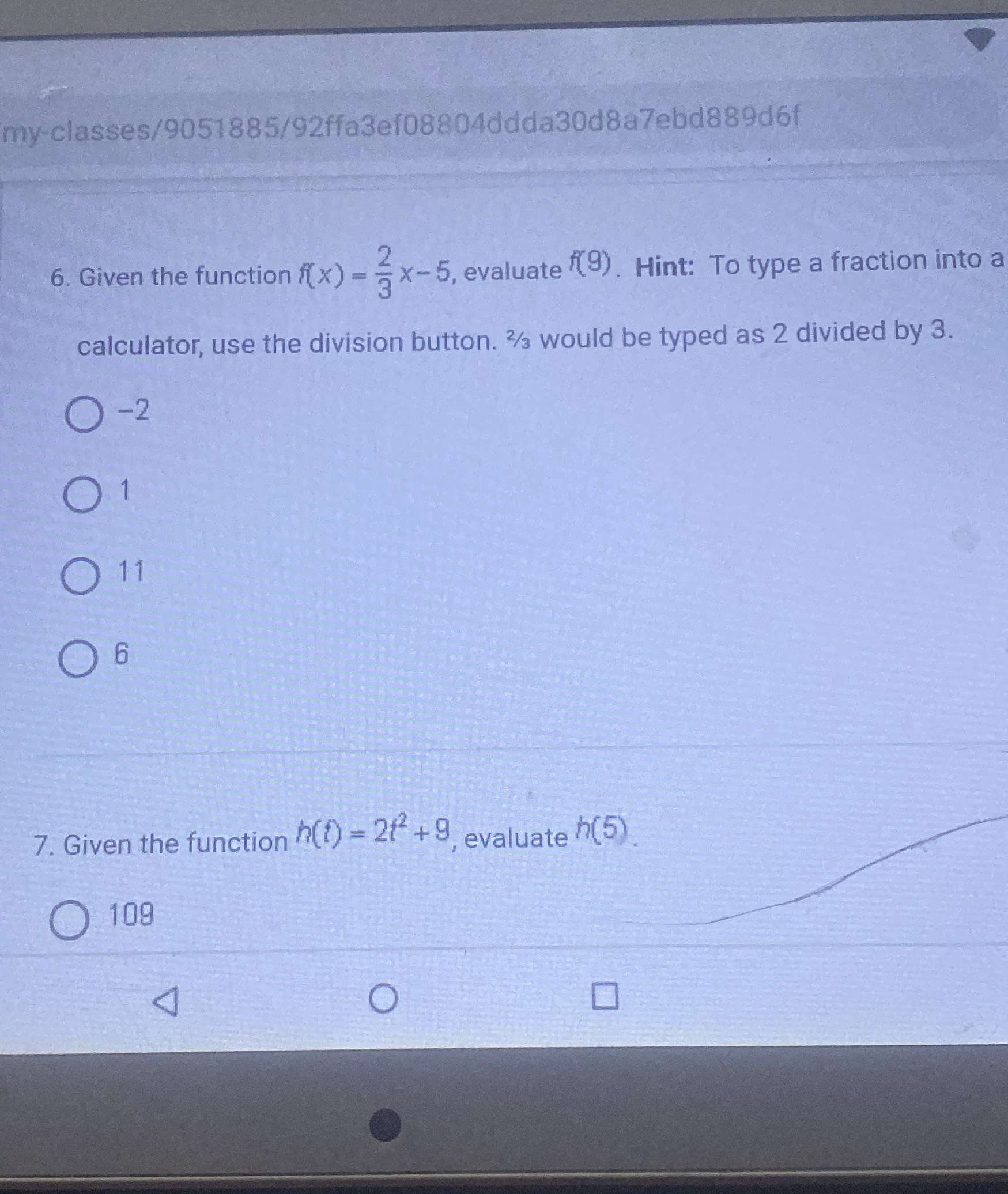### ¿Todavía tienes preguntas de matemáticas?

Pregunte a nuestros tutores expertos
Algebra
Pregunta6. Given the function $$f ( x ) = \frac { 2 } { 3 } x - 5$$ , evaluate $$f ( 9 )$$ . Hint: To type a fraction into a calculator, use the division button. $$2 / 3$$ would be typed as $$2$$ divided by $$3$$ .

$$- 2$$

$$1$$

$$11$$

$$6$$

7. Given the function $$h ( t ) = 2 t ^ { 2 } + 9$$ , evaluate $$h ( 5 )$$ .